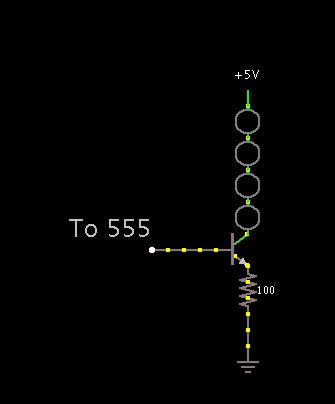# Hello i want to know if my circuit would work in real life

circuit_1.png (7671Bytes)

hello
im building a flashing heart for my girlfriend, im using a 555 chip and 7 LEDs

i have a circuit lay out from multisim it says it will work but i have my doubts

i wanted the potentiometer to change how fast the LEDS flash on  multisim  its always the same soo im not sure why

im using 10 mm LEDs

you will not need

the trasistors if you use small LEDs.

circuit

circuit 2This circuit won’t work with 5V

A typical red LED drops 1.8V, and the NPN transistor itself will drop some voltage too. So the 5V you show won’t be enough.

You can run a 555 timer at voltage from about 4.5V to 15V. So using this circuit may work at a higher voltage.

There’s a good page on the 555 timer here.

Read the section on astable operation, in particular the bit about choosing R1, R2, and C1 (referring to their schematic, not to yours). You can replace R2 with a variable resistor.

From what I can tell the

From what I can tell the circuit would work, however it is not necessarry to drive each LED individually via a transistor. I would drive a couple LED’s from each transistor. Don’t forget the correct resistance for the transistor to avoid pulling too much current.

this should work betterTake a read through this post: How to make LEDs glow, not blow.

Be careful. In the diagram above, you now have the current for all five LEDs flowing through one current limiting resistor. You have to adjust the value of the resistor.

Let’s say you want each LED to run at 25mA. So your target current through the resistor and transistor is:

5 x 0.025 = 0.125A (125mA)

Let’s say the voltage drop through the transistor from collector to emitter is 0.6V, and we’ll stick with 1.8V drop for the LEDs (You need to look up the actual voltage drop for your LEDs.)  To determine the voltage drop across the resistor, we subtract these two numbers from the supply voltage:

5 - 0.6 - 1.8 = 2.6V

To determine the correct value of the resistor, use Ohm’s Law (V = IR), solving for R:

R = V / I = 2.6V / 0.125A = 20.8 Ohms.

Be sure that your resistor can handle the current. I’d go with a 1/2 Watt resistor to be safe.

BTW, you won’t find a 20.8 Ohm resistor. Just use the closest value you can find. You can re-run the calucations above to be sure you know what current to actually expect.

Thanks!

I am not very good at calculating the resistors. i just use 100-1k ohm resistors depending on the led and voltage.